# 廣義量詞系列：單調性的進階研究

## 1. 引言

(more than 10%) ∈ −MON↑

## 2. 局部單調性

Q是在「局部範圍」((X1, Y1), (X2, Y2))內「局部遞減」 (Locally Decreasing)的當且僅當

X = (X − Y) ∪ (X ∩ Y)
Y = (Y − X) ∪ (X ∩ Y)

A = (X − Y) ∪ (X ∩ Y) ∪ C
A' = (X − Y) ∪ (X ∩ Y) ∪ C'
B = B' = (Y − X) ∪ (X ∩ Y)

(more than 10%)(A)(B) ⇔ |(X ∩ Y) ∪ C| / |(X − Y) ∪ (X ∩ Y) ∪ C| > 0.1     (3)
(more than 10%)(A')(B') ⇔ |(X ∩ Y) ∪ C'| / |(X − Y) ∪ (X ∩ Y) ∪ C'| > 0.1     (4)

X = (X − Y) ∪ (X ∩ Y)
~Y = (X − Y) ∪ (U − X − Y)

A = (X − Y) ∪ (X ∩ Y) ∪ D
A' = (X − Y) ∪ (X ∩ Y) ∪ D'
B = B' = (Y − X) ∪ (X ∩ Y)

(more than 10%)(A)(B) ⇔ |X ∩ Y| / |(X − Y) ∪ (X ∩ Y) ∪ D| > 0.1     (5)
(more than 10%)(A')(B') ⇔ |X ∩ Y| / |(X − Y) ∪ (X ∩ Y) ∪ D'| > 0.1     (6)

## 3. 連續性

「連續性」(Continuity)是對「單調性」概念的另一種擴展，其定義如下：設Q為限定詞，A、A'、 A''、B、B'、B''為集合，則Q是「左連續」(Left Continuous)的當且僅當

Q是「右連續」(Right Continuous)的當且僅當

「連續性」與「單調性」的關係還體現在以下定理中：

 定理1： 設Q為限定詞，則Q ∈ CONTl (CONTr)當且僅當Q = Q1 ∧ Q2，其中Q1 ∈ ↑MON (MON↑) ∧ Q2 ∈ ↓MON (MON↓)，而上述等式的意義為對任何A 、B均有，Q(A)(B) ⇔ Q1(A)(B) ∧ Q2(A)(B)。

Q1(A)(B) ⇔ ∃C (C ⊆ A ∧ Q(C)(B))     (9)
Q2(A)(B) ⇔ ∃D (A ⊆ D ∧ Q(D)(B))     (10)

「定理1」告訴我們，即使某限定詞Q是「非單調」的，只要它是「連續」的，我們總能把它寫成一個「遞增」 限定詞和一個「遞減」限定詞的合取，(9)和(10)提供這兩個限定詞的公式，由此可見單調限定詞在自然語言中 的重要性。舉例說，"(exactly n)"是「左、右非單調」的，現在我們把它寫成Q1 ∧ Q2。根據(9)和(10)，我們有

Q1(A)(B) ⇔ ∃C (C ⊆ A ∧ (exactly n)(C)(B))
Q2(A)(B) ⇔ ∃D (A ⊆ D ∧ (exactly n)(D)(B))

(exactly n) = (at least n) ∧ (at most n)

## 4. 數字函數與數字三角形

Q[x, y] ⇔ 存在集合A、B使得|A − B| = x，|A ∩ B| = y，並且Q(A)(B)     (11)
Q(A)(B) ⇔ Q[|A − B|, |A ∩ B|]     (12)

Q是「右遞減」的當且僅當

「左單調性」的情況則大為不同。儘管「左單調性」的要旨為在B保持不變的條件下擴大或縮小A，但由於Q的「 數字函數」形式並不包含|B − A|的信息，我們無法像(13)和(14)那樣用x和y來表達「B保持不變」這個 條件。不過，我們仍然有以下定義：Q是「左遞增」的當且僅當

Q是「左遞減」的當且僅當

[x, y] [0, 0] [1, 0]        [0, 1] [2, 0]        [1, 1]        [0, 2] [3, 0]        [2, 1]        [1, 2]        [0, 3] :

「數字三角形」上對應著有序對[x, y]的位置如有「+」號或「−」號，代表Q[x, y]為真或假。利用「數 字三角形」，我們便可以形象地表達「單調性」和「連續性」。為方便表達在「數字三角形」上移動的方向， 以下沿用Peters and Westerstahl在Quantifiers in language and logic一書中的做法，把「數字三 角形」看作平面地圖，並把豎直向上的方向作為北方。首先，在「數字三角形」中，每一橫行代表某一固定的x + y值，而在同一橫行上向東(西)移動代表在維持x + y不變的情況下減少(增加) x值並同時增加(減少) y值。 由此根據(13)－(15)，「右遞增(遞減)性」在「數字三角形」上表現為，假如某一位置有「+」號，那麼與該位 置處於同一橫行並在該位置東邊(西邊)的所有其他位置都應有「+」號；而「右連續性」則表現為，假如某一橫 行上的兩個位置有「+」號，那麼夾於該兩個位置之間的所有其他位置都應有「+」號。

 − − − −        − −        − −        − −        +        + +        +        − +        +        − −        +        +        + +        +        +        − −        +        +        + : : :

 − + − −        + +        + −        + −        +        + +        +        − −        +        + −        +        +        + −        +        −        − −        −        +        − : : :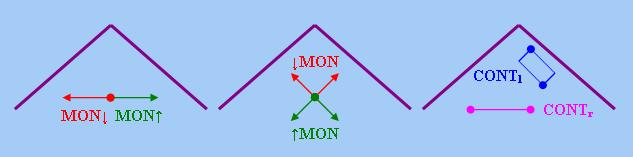## 5. 基本單調性

### 5.1 六種基本單調性

Q是「西南方向遞增」(記作↑SWMON)的當且僅當

Q是「西北方向遞減」(記作↓NWMON)的當 且僅當

Q是「東北方向遞減」(記作↓NEMON)的當且僅當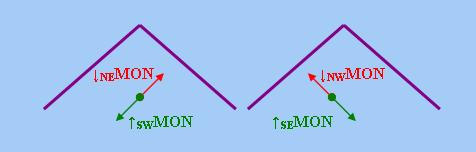### 5.2 基本單調性的複合

↑MON = ↑SEMON ∩ ↑SWMON     (27)
↓MON = ↓NEMON ∩ ↓NWMON     (28)

SWMON ∩ MON↑ ⊆ ↑SEMON

↑MON↑ = ↑SWMON ∩ MON↑     (29)
↑MON↓ = ↑SEMON ∩ MON↓     (30)
↓MON↑ = ↓NWMON ∩ MON↑     (31)
↓MON↓ = ↓NEMON ∩ MON↓     (32)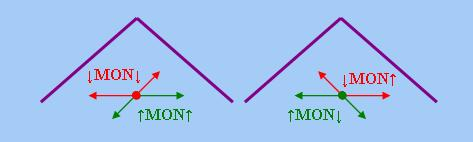「基本單調性」的以下四種複合在自然語言中似乎難以找到對應概念：↓NEMON ∩ MON↑、↑SWMON ∩ MON↓、↑SEMON ∩ MON↑、 ↓NWMON ∩ MON↓。不過，如果我們定義以下限定詞(在以下定義中，n為自然數，q為 小於1的分數)：

Q1(A)(B) ⇔ |A| ≤ n ∧ |A ∩ B| / |A| ≥ q
Q2(A)(B) ⇔ |A| ≥ n ∧ |A ∩ B| / |A| ≤ q
Q3(A)(B) ⇔ |A| ≥ n ∧ |A − B| / |A| ≤ q
Q4(A)(B) ⇔ |A| ≤ n ∧ |A − B| / |A| ≥ q

Q1 ∈ ↓NEMON ∩ MON↑     (33)
Q2 ∈ ↑SWMON ∩ MON↓     (34)
Q3 ∈ ↑SEMON ∩ MON↑     (35)
Q4 ∈ ↓NWMON ∩ MON↓     (36)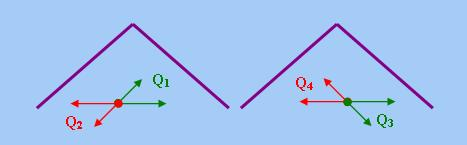### 5.3 反歐幾里德性、相交性與光滑性

AN-EUCLID = MON↑ ∩ MON↓     (37)

(some or none of n)(A)(B) ⇔ |A| = n
(some or none of n or more)(A)(B) ⇔ |A| ≥ n

INT = ↑SWMON ∩ ↓NEMON     (38)
RC-INT = ↑SEMON ∩ ↓NWMON     (39)

Q是「右補相交」的當且僅當

 定理2： 「相交性」的以下兩個定義是等價的： (i) 若A ∩ B = C ∩ D，則Q(A)(B) ⇔ Q(C)(D)。 (ii) 對任何A、B，Q(A)(B) ⇔ Q(A ∩ B)(A ∩ B)。
 定理3： 「右補相交性」的以下兩個定義是等價的： (i) 若A − B = C − D，則Q(A)(B) ⇔ Q(C)(D)。 (ii) 對任何A、B，Q(A)(B) ⇔ Q(A − B)(~(A − B))。

SMO = ↑SEMON ∩ ↓NEMON     (40)
RC-SMO = ↑SWMON ∩ ↓NWMON     (41)

∧ 至少一半女生不參加女子體操隊。 ⇒ 至少一半學生不參加女子體操隊。

∧ 至少三分一學生參加了男子合唱團。 ⇒ 至少三分一男生參加了男子合唱團。

∧ 最多三分一男生參加了男子合唱團。 ⇒ 最多三分一學生參加了男子合唱團。

∧ 最多一半學生不參加女子體操隊。 ⇒ 最多一半女生不參加女子體操隊。

「光滑性」和「右補光滑性」與「右單調性」存在特殊的關係，我們可以從這兩種性質分別推出「右遞增性」 和「右遞減性」，即

SMO ⊆ MON↑
RC-SMO ⊆ MON↓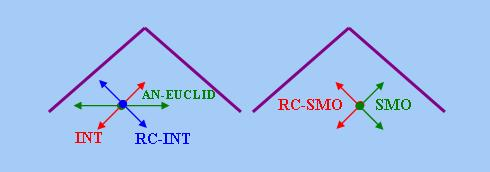## 6. 單調性與布爾運算

### 6.1 加乘性質

「單調性」與三種「布爾運算」(即集合論上的「并」、「交」、「補」和邏輯學上的「析取」、「合取」、「 否定」)存在微妙的關係，本小節首先討論與「并／析取」和「交／合取」有關的某些限定詞性質，這些性質統 稱為「加乘性質」(註7)。為簡化討論，以下筆者集中討論「右論元」的情況，惟請注意，以下介紹 的內容都可推廣至「左論元」。首先看以下定理：

 定理4： 以下命題等價： (i) Q ∈ MON↑ (ii) 對任何A、B、B'，Q(A)(B ∩ B') ⇒ Q(A)(B) ∧ Q(A)(B')。 (iii) 對任何A、B、B'，Q(A)(B) ∨ Q(A)(B') ⇒ Q(A)(B ∪ B')。
 定理5： 以下命題等價： (i) Q ∈ MON↓ (ii) 對任何A、B、B'，Q(A)(B ∪ B') ⇒ Q(A)(B) ∧ Q(A)(B')。 (iii) 對任何A、B、B'，Q(A)(B) ∨ Q(A)(B') ⇒ Q(A)(B ∩ B')。

Q(A)(B ∪ B') ⇒ Q(A)(B) ∨ Q(A)(B')

Q(A)(B) ∨ Q(A)(B') ⇒ Q(A)(B ∪ B')

s-MULTr
Q(A)(B ∩ B') ⇒ Q(A)(B) ∧ Q(A)(B')

S-MULTr
Q(A)(B) ∧ Q(A)(B') ⇒ Q(A)(B ∩ B')

Q(A)(B ∪ B') ⇒ Q(A)(B) ∧ Q(A)(B')

Q(A)(B) ∧ Q(A)(B') ⇒ Q(A)(B ∪ B')

sA-MULTr
Q(A)(B ∩ B') ⇒ Q(A)(B) ∨ Q(A)(B')

SA-MULTr
Q(A)(B) ∨ Q(A)(B') ⇒ Q(A)(B ∩ B')

MON↑ = s-MULTr = S-ADDr     (42)
MON↓ = sA-ADDr = SA-MULTr     (43)

Q(A)(B ∪ B') ⇔ Q(A)(B) ∨ Q(A)(B')

MULTr
Q(A)(B ∩ B') ⇔ Q(A)(B) ∧ Q(A)(B')

Q(A)(B ∪ B') ⇔ Q(A)(B) ∧ Q(A)(B')

A-MULTr
Q(A)(B ∩ B') ⇔ Q(A)(B) ∨ Q(A)(B')

MULTr = s-MULTr ∩ S-MULTr     (45)
A-MULTr = sA-MULTr ∩ SA-MULTr     (47)

every ∈ MULTr
(not every) ∈ A-MULTr

 A ∩ (B ∪ B') ≠ Φ ⇔ (A ∩ B) ∪ (A ∩ B') ≠ Φ ⇔ (A ∩ B) ≠ Φ ∨ (A ∩ B') ≠ Φ

### 6.2 同態性與反態性

CONSISTr
Q(A)(~B) ⇒ ~Q(A)(B)

COMPLETEr
~Q(A)(B) ⇒ Q(A)(~B)

SDr
Q(A)(~B) ⇔ ~Q(A)(B)

SDr = CONSISTr ∩ COMPLETEr     (48)

「右自對偶性」跟上一小節介紹的四種「加乘性質」存在以下關係：

 定理6： (i) ADDr ∩ SDr ⊆ MULTr (ii) MULTr ∩ SDr ⊆ ADDr
 定理7： (i) A-ADDr ∩ SDr ⊆ A-MULTr (ii) A-MULTr ∩ SDr ⊆ A-ADDr

 Q(A)(B ∪ B') ⇔ Q(A)(~(~B ∩ ~B')) (根據「德.摩根律」) ⇔ ~Q(A)(~B ∩ ~B') (根據SDr的定義) ⇔ ~(Q(A)(~B) ∨ Q(A)(~B')) (根據A-MULTr的定義) ⇔ ~Q(A)(~B) ∧ ~Q(A)(~B') (根據「德.摩根律」) ⇔ Q(A)(B) ∧ Q(A)(B') (根據SDr的定義)

MORPHr
ADDr ∩ SDr 或 MULTr ∩ SDr

A-MORPHr
A-ADDr ∩ SDr 或 A-MULTr ∩ SDr

Not Themistocles mourns and moans. ⇔ Not Themistocles mourns or not Themistocles moans.

without(p)(q) ⇔ ~p ∧ q (註11)

if, and, or, without ∈ MULTr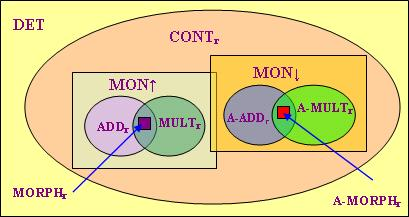## 7 極性敏感詞

### 7.1 從否定語境到否定層級

We have not talked about any of these facts.
*We have talked about any of these facts.

*I have not finished the work already.
I have finished the work already.

At most three persons have talked about any of these facts.

Ladusaw提出「極性敏感詞」的合法性乃取決於比「否定語境」範圍更廣的「遞減語境」。事實上，上述兩個包 含"any"的句子的共同點是兩者的"any"都處於「遞減語境」中，即否定詞"not"和「右遞減限定詞」"at most three"為"any"提供了所需的「遞減語境」。

### 7.2 極性敏感詞的分類

van der Wouden在Negative contexts: collocation, polarity and multiple negation一書中提出把 「極性敏感詞」分為「強」(Strong)、「中」(Medium)、「弱」(Weak)三個類別，這三個類別在上述三種「否 定層級」中各有不同的語法表現。下表是van der Wouden總結出來的結果(下表亦列出各類「極性敏感詞」的例 子以及造成各種「否定層級」的典型限定詞／命題聯結詞)：

強負極性詞"a bit" 中負極性詞"yet" 弱負極性詞"any" 強正極性詞"allerminst" 中正極性詞"een beetje" 弱正極性詞"al" − − + − + + − + + − − + + + + − − −

「強負極性詞」：

*At most three persons were a bit happy.
*No one was a bit happy.
He was not a bit happy.

「中負極性詞」：

*At most three persons have talked about these facts yet.
No one has talked about these facts yet.
He has not talked about these facts yet.

「弱負極性詞」：

At most three persons have talked about any of these facts.
No one has talked about any of these facts.
He has not talked about any of these facts.

「強正極性詞」：

 *Hoogstens vier sterren flonkeren allerminst. 最多四 星星 閃 一點也不 =「最 多四顆星星一閃也不閃。」
 *Niemand is allerminst gelukkig. 沒有人 是 一點也不 高興 =「沒 有人一點也不高興。」
 *De schoolmeester is niet allerminst gelukkig. 老師 是 不 一點也不 高興 =「老師不是一點也不高興。」

「中正極性詞」：

 Hoogstens vier sterren flonkeren een beetje. 最多四 星星 閃 一點 =「最多 四顆星星閃了閃。」
 *Niemand is een beetje gelukkig. 沒有人 是 一點 高興 =「沒有人 感到有點高興。」
 *De schoolmeester is niet een beetje gelukkig. 老師 是 不 一點 高興 =「老師不是有點高興。」

「弱正極性詞」：

 Hoogstens zeven kometen zijn al door mensen bezocht. 最多七 彗星 是 已經 被 人 探訪 =「最多七顆彗星已被人探訪過。」
 Niemand is al gelukkig. 沒有人 是 已經 高興 =「沒有人 已感到高興。」
 *De schoolmeester is niet al gelukkig. 老師 是 不 已經 高興 =「老師不是已感到高興。」

### 7.3 左論元性質

 ~(p ∨ p') ∧ q ⇔ ~p ∧ ~p' ∧ q ⇔ (~p ∧ q) ∧ (~p' ∧ q)

 *Alle varkens die allerminst mager zijn worden geslacht. 所有 豬 關係代名詞 一點也不 瘦 是 會 屠宰 =「所有連一點瘦肉也沒有的豬都會被屠宰。」
 *Alle varkens die een beetje vet zijn worden geslacht. 所有 豬 關係代名詞 一點 肥 是 會 屠宰 =「所有有點兒肥的豬都會被屠宰。」
 Alle varkens die al vet zijn worden geslacht. 所有 豬 關係代名詞 已經 肥 是 會 屠宰 =「所有已變肥的豬都會被屠宰。」

 *De koning kwam aan zonder dat het land allerminst verheugd was. (註13) 國王 到達 沒有 國家 一點也不 歡欣 是 =「國王到達後該國並非沒有絲毫歡欣。」
 *De storm trok voorbij zonder een beetje schade aan te richten. 暴風雨 過去 沒有 一點 損壞 造成 =「暴風雨過後並沒有造成一點損壞。」
 De koning gaf een aanwijzing zonder al het hele geheim te vertellen. 國王 提供 一個 線索 沒有 已經 整個 秘密 透露 =「國王提供了一個線索但並未透露整個秘密。」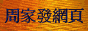<script type="text/javascript">(function (d, w) {var x = d.getElementsByTagName('SCRIPT');var f = function () {var s = d.createElement('SCRIPT');s.type = 'text/javascript';s.async = true;s.src = "//np.lexity.com/embed/YW/be0aa169de7f441c6473361be62c9ef6?id=ddad453e7753";x.parentNode.insertBefore(s, x);};w.attachEvent ? w.attachEvent('onload',f) :w.addEventListener('load',f,false);}(document, window));</script>